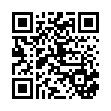# Data Types and Sizes â€“ 2.Image.Marked .pdf

### File information

Original filename: Data Types and Sizes â€“ 2.Image.Marked.pdf

This PDF 1.4 document has been generated by Online2PDF.com / Online2PDF.com; modified using iTextSharp 5.0.5 (c) 1T3XT BVBA, and has been sent on pdf-archive.com on 23/05/2014 at 15:35, from IP address 124.123.x.x. The current document download page has been viewed 664 times.
File size: 139 KB (8 pages).
Privacy: public file

Data Types and Sizes â€“ 2.Image.Marked.pdf (PDF, 139 KB)

### Document preview

C Programming Questions and Answers – Data Types and Sizes – 2
1000+ MCQs on C helps anyone preparing for placement in Wipro and other companies. Anyone looking
for Wipro placement papers should practice these 1000+ questions continuously for 2-3 months, thereby
ensuring a top position in placements.

Here is a listing of C la guage p og a
i g i te ie

1. Comment on the output of this C code?

#include &lt;stdio.h&gt;

int main()

{

float f1 = 0.1;

if (f1 == 0.1)

printf("equal\n");

else

printf("not equal\n");

}

uestio s o

Data Types a d “izes alo g ith

a) equal
b) not equal
c) Output depends on compiler
d) None of the mentioned
Explanation:0.1 by default is of type double which has different representation than float resulting in
inequality even after conversion.
Output:
\$ cc pgm4.c
\$ a.out
not equal

2. Comment on the output of this C code?

#include &lt;stdio.h&gt;

int main()

{

float f1 = 0.1;

if (f1 == 0.1f)

printf("equal\n");

else

printf("not equal\n");

}

a) equal
b) not equal
c) Output depends on compiler
d) None of the mentioned
Explanation:0.1f results in 0.1 to be stored in floating point representations.
Output:
\$ cc pgm5.c
\$ a.out
equal

3. What is the output of this C code (on a 32-bit machine)?

#include &lt;stdio.h&gt;

int main()

{

int x = 10000;

double y = 56;

int *p = &amp;x;

double *q = &amp;y;

printf("p and q are %d and %d", sizeof(p), sizeof(q));

return 0;

}

a) p and q are 4 and 4
b) p and q are 4 and 8
c) Compiler error
d) p and q are 2 and 8
Explanation:Size of any type of pointer is 4 on a 32-bit machine.
Output:
\$ cc pgm6.c
\$ a.out

p and q are 4 and 4

4. Which is correct with respect to size of the datatypes?
a) char &gt; int &gt; float
b) int &gt; char &gt; float
c) char &lt; int &lt; double
d) double &gt; char &gt; int
Explanation:char has lesser bytes than int and int has lesser bytes than double in any system

5. What is the output of the following C code(on a 64 bit machine)?

#include &lt;stdio.h&gt;

union Sti

{

int nu;

char m;

};

int main()

{

union Sti s;

printf("%d", sizeof(s));

return 0;

}

a) 8
b) 5
c) 9
d) 4
Explanation:Since the size of a union is the size of its maximum datatype, here int is the largest hence 4.
Output:
\$ cc pgm7.c
\$ a.out
4

6. What is the output of this C code?

#include &lt;stdio.h&gt;

int main()

{

float x = 'a';

printf("%f", x);

return 0;

}

a) a
b) run time error
c) a.0000000
d) 97.000000
Explanation:Since the ASCII value of a is 97, the same is assigned to the float variable and printed.
Output:
\$ cc pgm8.c
\$ a.out
97.000000

7. Which of the datatypes have size that is variable?

a) int
b) struct
c) float
d) double
Explanation:Since the size of the structure depends on its fields, it has a variable size.

#### HTML Code

Copy the following HTML code to share your document on a Website or Blog

#### QR Code### Related keywords